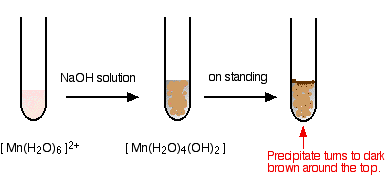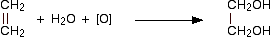# Chemistry of Manganese

$$\newcommand{\vecs}{\overset { \rightharpoonup} {\mathbf{#1}} }$$ $$\newcommand{\vecd}{\overset{-\!-\!\rightharpoonup}{\vphantom{a}\smash {#1}}}$$$$\newcommand{\id}{\mathrm{id}}$$ $$\newcommand{\Span}{\mathrm{span}}$$ $$\newcommand{\kernel}{\mathrm{null}\,}$$ $$\newcommand{\range}{\mathrm{range}\,}$$ $$\newcommand{\RealPart}{\mathrm{Re}}$$ $$\newcommand{\ImaginaryPart}{\mathrm{Im}}$$ $$\newcommand{\Argument}{\mathrm{Arg}}$$ $$\newcommand{\norm}{\| #1 \|}$$ $$\newcommand{\inner}{\langle #1, #2 \rangle}$$ $$\newcommand{\Span}{\mathrm{span}}$$ $$\newcommand{\id}{\mathrm{id}}$$ $$\newcommand{\Span}{\mathrm{span}}$$ $$\newcommand{\kernel}{\mathrm{null}\,}$$ $$\newcommand{\range}{\mathrm{range}\,}$$ $$\newcommand{\RealPart}{\mathrm{Re}}$$ $$\newcommand{\ImaginaryPart}{\mathrm{Im}}$$ $$\newcommand{\Argument}{\mathrm{Arg}}$$ $$\newcommand{\norm}{\| #1 \|}$$ $$\newcommand{\inner}{\langle #1, #2 \rangle}$$ $$\newcommand{\Span}{\mathrm{span}}$$$$\newcommand{\AA}{\unicode[.8,0]{x212B}}$$

This page looks at some aspects of manganese chemistry. It includes: two simple reactions of manganese(II) ions in solution (summarized from elsewhere on the site), and the use of potassium manganate(VII) (potassium permanganate) as an oxidizing agent - including its use in titrations. The simplest ion that manganese forms in solution is the hexaaquamanganese(II) ion - $$[Mn(H_2O)_6]^{2+}$$.

### The reaction of hexaaquamanganese(II) ions with hydroxide ions

Hydroxide ions (from, say, sodium hydroxide solution) remove hydrogen ions from the water ligands attached to the manganese ion. Once a hydrogen ion has been removed from two of the water molecules, you are left with a complex with no charge - a neutral complex. This is insoluble in water and a precipitate is formed.

$[Mn(H_2O)_6]^{2+} + 2OH^- \rightarrow [Mn(H_2O)_4(OH)_2] + 2H2O \nonumber$

In the test-tube, the color changes are:I have shown the original solution as very pale pink (the palest I can produce!), but in fact it is virtually colorless. The pale brown precipitate is oxidized to darker brown manganese(III) oxide in contact with oxygen from the air.

### The reaction of hexaaquamanganese(II) ions with ammonia solution

Ammonia can act as both a base and a ligand. In this case, at usual lab concentrations, it simply acts as a base - removing hydrogen ions from the aqua complex.

$[Mn(H_2O)_6]^{2+} + 2NH_3 \rightarrow [Mn(H_2O)_4(OH)_2] + 2NH_4^+ \nonumber$Again, I have shown the original solution as the palest pink I can produce, but in fact it is virtually colorless. The pale brown precipitate is oxidized to darker brown manganese(III) oxide in contact with oxygen from the air. There is no observable difference in appearance between this reaction and the last one.

## Potassium manganate(VII) chemistry

Potassium manganate(VII) (potassium permanganate) is a powerful oxidizing agent.

### Using potassium manganate(VII) as an oxidizing agent in organic chemistry

Potassium manganate(VII) is usually used in neutral or alkaline solution in organic chemistry. Acidified potassium manganate(VII) tends to be a rather destructively strong oxidizing agent, breaking carbon-carbon bonds. The potassium manganate(VII) solution is usually made mildly alkaline with sodium carbonate solution, and the typical colour changes are:### In testing for a C=C double bond

Potassium manganate(VII) oxidizes carbon-carbon double bonds, and so goes through the colour changes above. Ethene, for example, is oxidized to ethane-1,2-diol.The oxygen in square brackets is taken to mean "oxygen from an oxidizing agent". This abbreviated form of the equation is most commonly used in organic chemistry. You are very unlikely to have to write the complete ionic equation for this reaction at this level. This isn't a good test for a carbon-carbon double bond, because anything which is even mildly reducing would have the same effect on the potassium manganate(VII) solution. You could, however, use this reaction simply as a means of making the diol.

### In the oxidation of aromatic side chains

Alkaline potassium manganate(VII) solution oxidizes any hydrocarbon side chain attached to a benzene ring back to a single -COOH group. Prolonged heating is necessary. For example:In the case of the ethyl side chain, you will also get carbon dioxide. With longer side chains, you will get all sorts of mixtures of other products - but in each case, the main product will be benzoic acid.

### Using potassium manganate(VII) as an oxidizing agent in titrations

Potassium manganate(VII) solution is used to find the concentration of all sorts of reducing agents. It is always used in acidic solution. For example, it oxidizes

• iron(II) ions to iron(III) ions

$Fe^{2+} \rightarrow Fe_{2+} + e- \nonumber$

• hydrogen peroxide solution to oxygen

$H_2O_2 \rightarrow O_2 + 2H^+ 2e^- \nonumber$

• ethanedioic acid to carbon dioxide (This reaction has to be done hot.)• sulfite ions (sulphate(IV) ions) to sulfate ions (sulfate(VI) ions)

$SO_3^{2-} + H_2O \rightarrow SO_4^{2-} + 2H^+ + 2e^- \nonumber$

In each case, the half-equation for the manganate(VII) ions in acidic solution is:

$MnO_4^- + 8H^+ +5e^- \rightarrow Mn^{2+} + 4H_2O \nonumber$

These equations can be combined to give you an overall ionic equation for each possible reaction. That, of course, also gives you the reacting proportions. For example, when the equations are combined, you find that 1 mole of MnO4- ions react with 5 moles of Fe2+ ions. Having got that information, the titration calculations are just like any other ones.

### Doing the titration

The potassium manganate(VII) solution always goes into the burette, and the other solution in the flask is acidified with dilute sulfuric acid. As the potassium manganate(VII) solution is run into the flask it becomes colourless. The end point is the first faint trace of permanent pink in the solution showing that there is a tiny excess of manganate(VII) ions present.

##### Problems with the use of potassium manganate(VII) solution

There are two things you need to be aware of:

• Potassium manganate(VII) cannot be used in titrations in the presence of ions like chloride or bromide which it oxidizes. An unknown amount of the potassium manganate(VII) would be used in side reactions, and so the titration result would be inaccurate. This is why you don't acidify the solution with hydrochloric acid.
• Potassium manganate(VII) isn't a primary standard. That means that it cannot be made up to give a stable solution of accurately known concentration.
• It is so strongly colored that it is impossible to see when all the crystals you have used have dissolved, and over a period of time it oxidizes the water it is dissolved in to oxygen.
• Bottles of potassium manganate(VII) solution usually have a brown precipitate around the top. This is manganese(IV) oxide - and is produced when the manganate(VII) ions react with the water.
• You have to make up a solution which is approximately what you want, and then standardize it by doing a titration. This is often done with ethanedioic acid solution, because this is a primary standard.